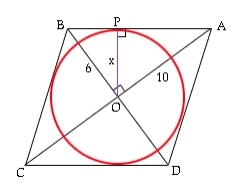# Area of circle inscribed within rhombus?

Circle inscribed in a rhombus touches its four side a four ends. The side of rhombus is a tangent to the circle.Here, r is the radius that is to be found using a and, the diagonals whose values are given.

Now the area of triangle AOB = ½ * OA * OB = ½ * AB * r (both using formula ½*b*h).

½ *a/2*b/2 = ½ *( √ (a2/4 + b2/4))*r

a*b/8 = √ (a2+ b2 )*r /4

r = a*b/ 2√ (a2+ b2 )

Area of circle = π*r*r = π*(a2*b2)/4(a2+ b2 )

## Example

The diagonal of the rhombus 5 & 10.

Area is 15.700000

## Example Code

Live Demo

#include <stdio.h>
int main(void) {
int a = 5; int b= 10;
float pie = 3.14;
float area = (float)((pie*a*a*b*b)/(4*((a*a)+(b*b))));
printf("The area of circle inscribed in the rhombus of diagonal %d and %d is %f",a,b,area);
return 0;
}

## Output

The area of circle inscribed in the rhombus of diagonal 5 and 10 is 15.700000